Search IntMath
Close

# IntMath Newsletter - WolframAlpha, point of inflection

By Murray Bourne, 30 May 2009

1. Math tip (a) - Studying for math exams
2. Math tip (b) - Wolfram Alpha
3. Math tip (c) - Point of Inflection and house prices
4. From the Math Blog
5. Final thought - who are your real friends?

## 1. Math tip (a) - Studying for math exams

Many of you are facing final math exams at this time of the year. Here are some articles that may help you:

Summer: And how will you use your summer (those of you in the Northern hemisphere)? I suggest that you consider doing a bit of math here and there during your holidays. You will beat the forgetting curve, which is very important for future math success.

## 2. Math tip (b) - Wolfram Alpha

WolframAlpha is a new online tool can show you how to do math — and much more.

It is a "computational knowledge engine", based on the Mathematica computer algebra system and it is one of the most significant recent online offerings.

Their aim is to

... make it possible to compute whatever can be computed about anything

Wolfram Alpha has a search function, but it has more math capabilities than Google's:

As of now, Wolfram|Alpha contains 10+ trillion of pieces of data, 50,000+ types of algorithms and models, and linguistic capabilities for 1000+ domains.

Those algorithms can help math students the most.

Enter something like "graph sin x" and it will provide you with 2 plots showing different domains (first from -2pi to +2pi and the other from -20 to 20.)

Another example: If you enter "x2 + 3x − 2", it will give you a wealth of useful information:

• The graph of the function (ie y = x2 + 3x − 2 using 2 different domains)
• A description of the curve ("a parabola")
• The roots of the expression (that is, the solution for x2 + 3x − 2 = 0)
• The derivative, indefinite integral and the minimum value
• And assorted other bits of information

Two plots of y = x2 + 3x − 2: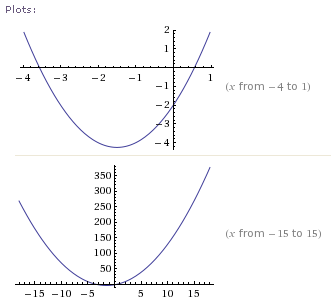The solutions for x2 + 3x − 2 = 0: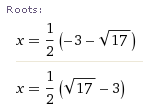Minimum value of the function: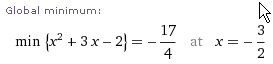Calculus of the function f(x) = x2 + 3x − 2: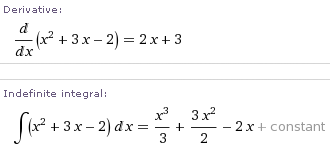It gives the steps for the integral of x2 + 3x − 2: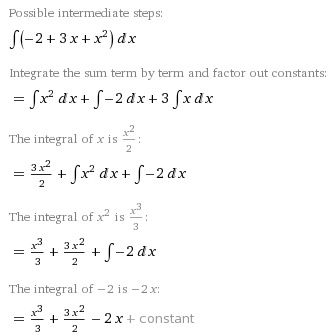Will it do my math homework for me? As long as you know what to ask Wolfram Alpha, there is a good chance it will do most of your homework.

But is that a good thing? This is a great tool but it can be dangerous. There is a good chance your math skills will deteriorate if you use this too much and begin to rely on it.

My suggestion is to do your homework questions first and later check your solutions using Wolfram Alpha.

That link again: WolframAlpha. Try some of their "sample inputs" when you get there.

## 3. Math tip (c) - Point of Inflection

Have we reached a "point of inflection" when it comes to house prices?In the UK it appears that house prices have bottomed, but not so in the USA yet.

In this special article for this Newsletter, we learn what a point of inflection really means and why it is important for your future job. It's also a good introduction to the concepts of maximum, minimum and rate of change graphs.

## 4. From the math blog

a) Will the DJIA follow the Nikkei down?
Will the DJIA follow Japan's Nikkei? The conditions are eerily similar to Japan's situation in the early 1990s.

b) Friday Math movie - Kinetic Wave Sculptures
Here's some kinetic (moving) art based on trigonometry.

The following 2 articles are mentioned above:

c) Point of inflection for house prices?
Have UK house prices reached a turn around? What does "point of inflection" mean, anyway?

d) Wolfram's Alpha - a computational knowledge engine
Here's a free online tool that will do algebra, calculus and trigonometry problems, as well as tell you what day of the week you were born!

## 5. Final Thought - who are your real friends?

Oprah Winfrey has inspired many people to live a better life. Her enthusiasm for reading, learning and teachers shows in everything she does. She has built a school in Africa and continually tries to help reduce the dropout rate in American schools.

This quote from Oprah is relevant for all students, especially math students:

Surround yourself with only people who are going to lift you higher.

Avoid the people who tell you math sucks, is useless and is a waste of time. They will only bring you down. Instead, surround yourself with people who love learning and are curious. Your life will be so much richer.

Until next time.

### Comment Preview

HTML: You can use simple tags like <b>, <a href="...">, etc.

To enter math, you can can either:

1. Use simple calculator-like input in the following format (surround your math in backticks, or qq on tablet or phone):
a^2 = sqrt(b^2 + c^2)
(See more on ASCIIMath syntax); or
2. Use simple LaTeX in the following format. Surround your math with $$ and $$.
$$\int g dx = \sqrt{\frac{a}{b}}$$
(This is standard simple LaTeX.)

NOTE: You can mix both types of math entry in your comment.